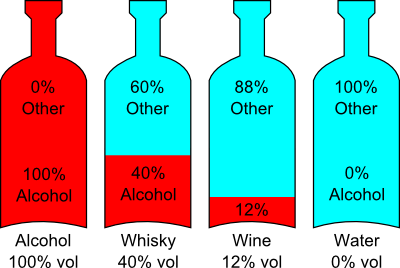ABV

Standing for 'Alcohol By Volume' this is the common measurement for describing how much alcohol is in a solution in the UK.

Seen on all alcoholic drink bottles in its more usual form '% vol', short for 'percentage of volume'.

It is a straight percentage telling the amount of alcohol. 100% vol means all of the solution is alcohol, 50% would mean half, 5% would be 1/20th etc.

For instance, if you drank 10 pints of beer at 5% vol that would be 10/20ths of a pint (Which is 1/2 a pint) of alcohol.
As 40% is less than half, if you drank a pint of whisky at 40% vol you would have drunk less alcohol than what is in 10 pints of beer at 5% vol.VWP SterilizerNever be caught outStock up NOW!